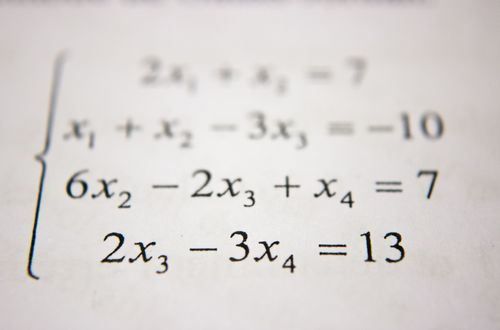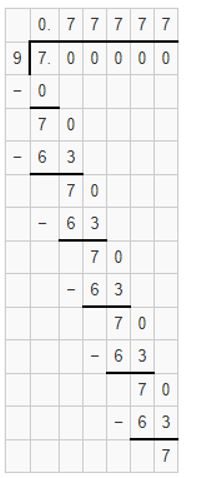# How to write recurring decimals

Want to find out how to write recurring decimals? We've provided a step-by-step guide of how to write recurring decimals to teach you everything you need to know.## Step 1: Divide the numerator by the denominator

To write a fraction to a recurring decimal, divide the numerator by the denominator. So, given the fraction in the form of a/b , find the recurring decimal using a÷b. This means that if we write 7/12 as a recurring decimal, we have

## Step 2: Use the - or .

If the digits are repeating, write “( ) ̅” or “( ) ̇” above the repeating digits. For the case of 7/12 , 3 is a repeating digit so we can write 7/12 as 0.583 ̅ or 0.583 ̇.

## Examples of how to write recurring decimals

**Q1) Write the fraction 7/9 as a recurring decimal. **

To change 7/9 as a recurring decimal, we divide 7 by 9.

7÷9 =0.777…

Since 7 is a repeating digit, we can mark 7 with “( ) ̅” or “( ) ̇” above it.

7/9 =0.7 ̅ or 0.7 ̇#### Join today

The fastest way to practice

Unlock our complete testing platform and improve faster that ever.